# RD Sharma Solutions Class 12 Area Bounded Regions Exercise 21.4

RD Sharma Solutions for Class 12 Maths Exercise 21.4 Chapter 21 Areas of Bounded Regions is the most preferred study material due to its unique description of the concepts. In this RD Sharma Solutions for Class 12 Maths Chapter 21, a distinctive attempt is made to build an understanding of the problems. Pursuing this chapter would ensure that you develop a piece of in-depth knowledge about the steps and methods of solving problems.

## Download PDF of RD Sharma Solutions for Class 12 Maths Chapter 21 Exercise 4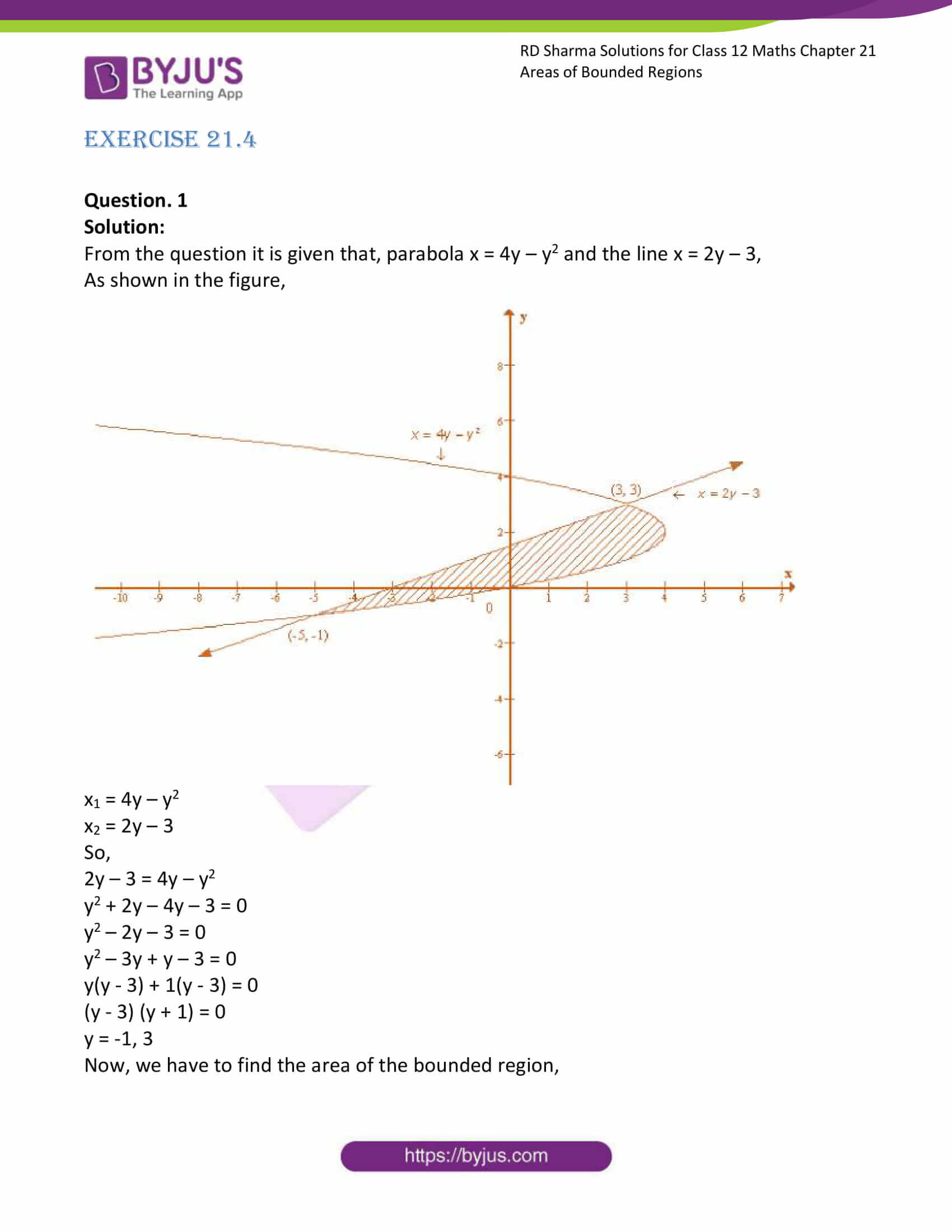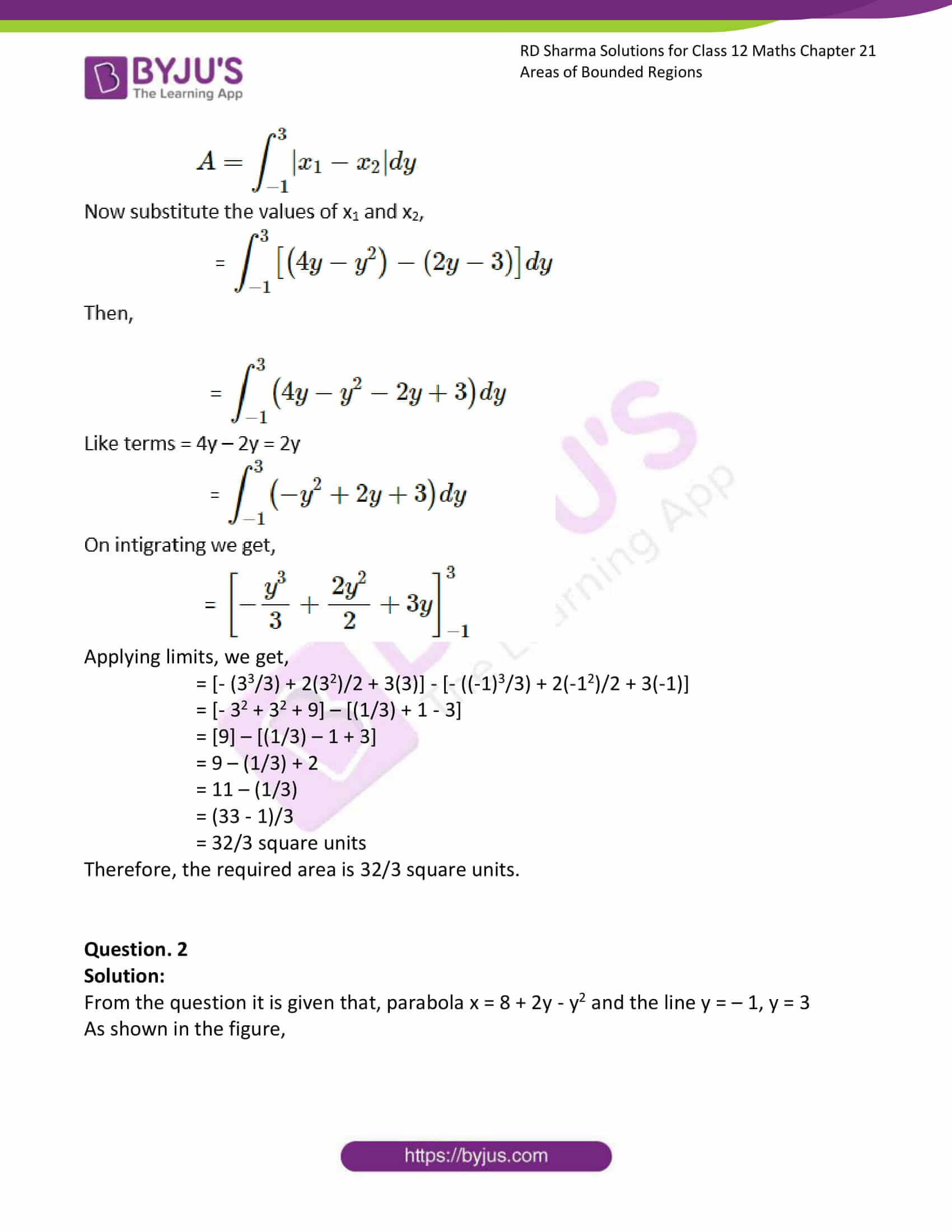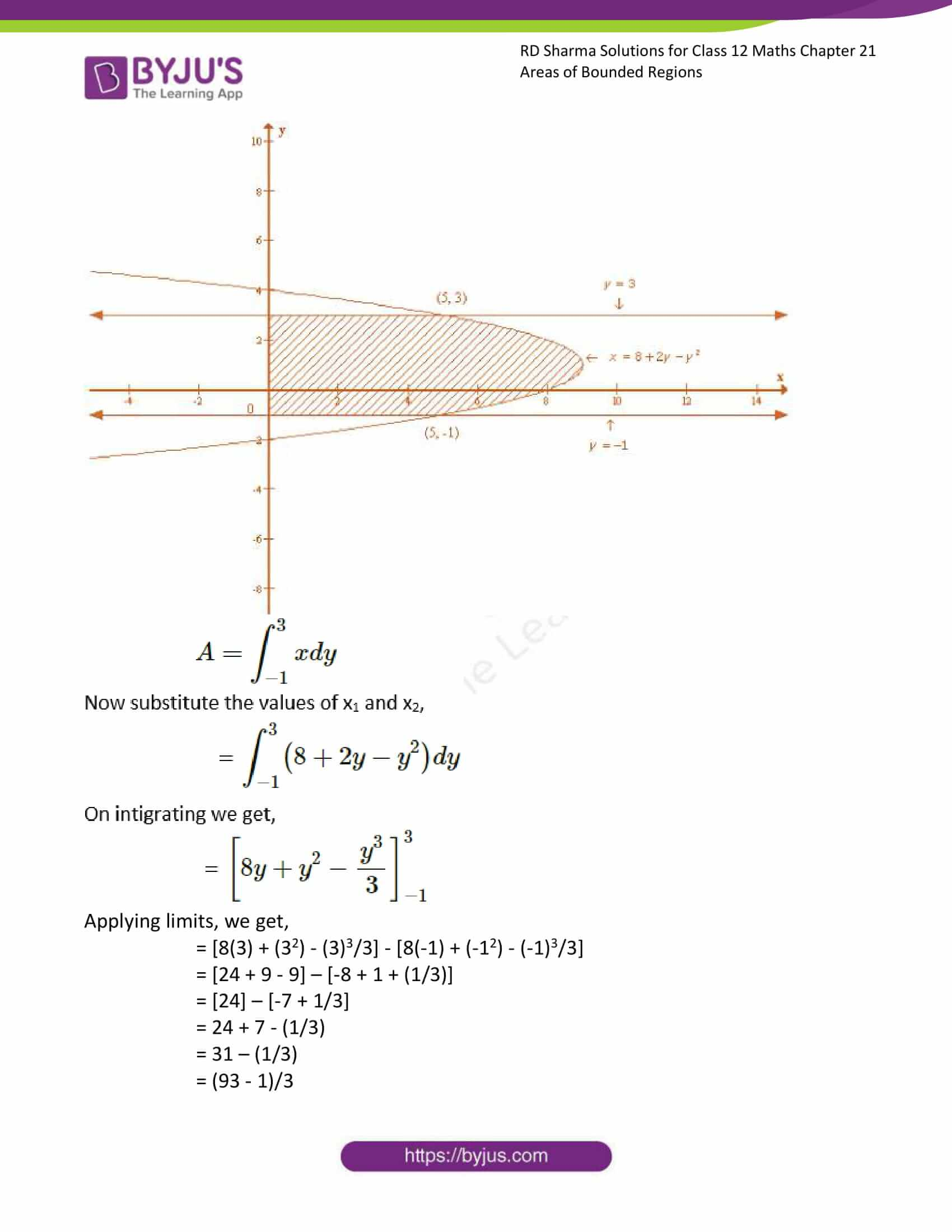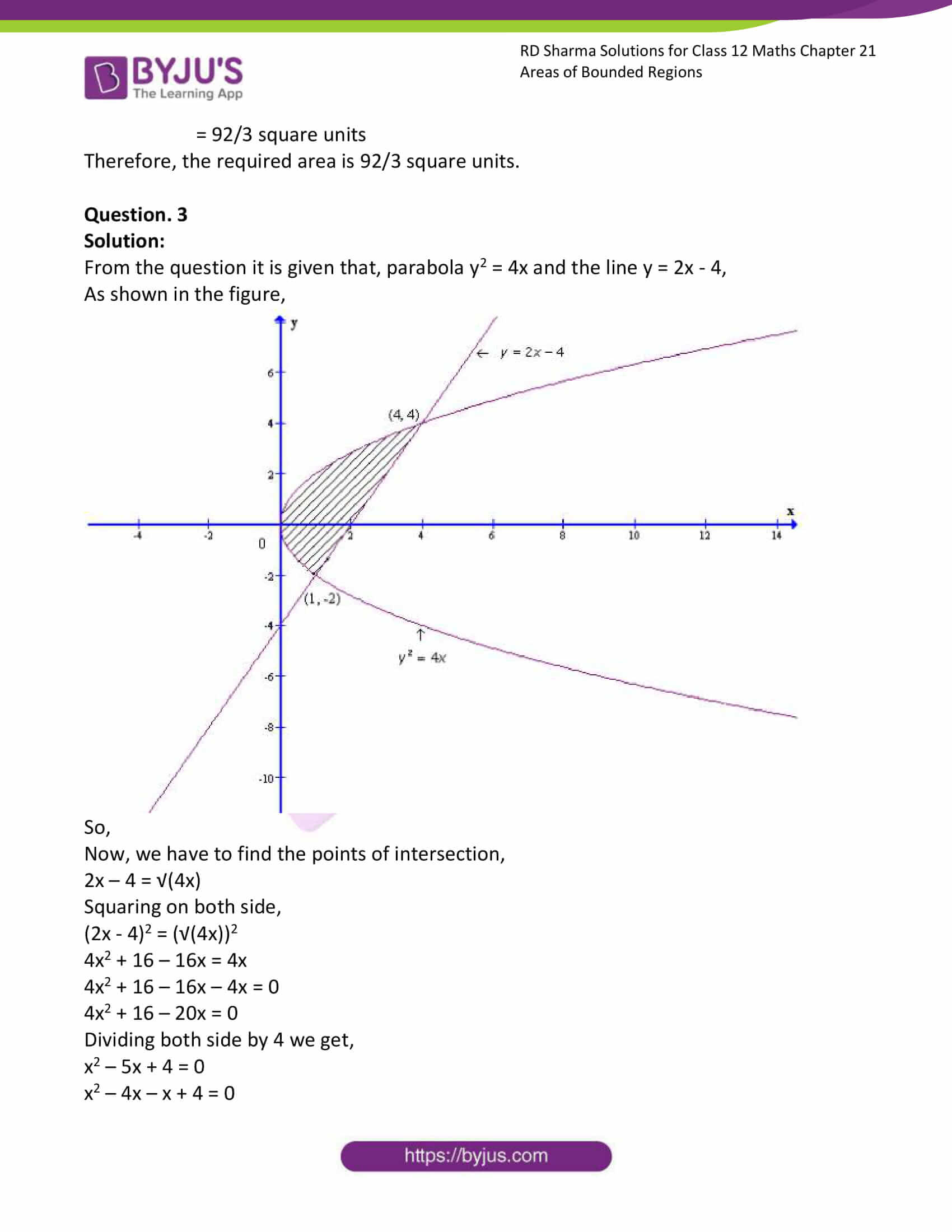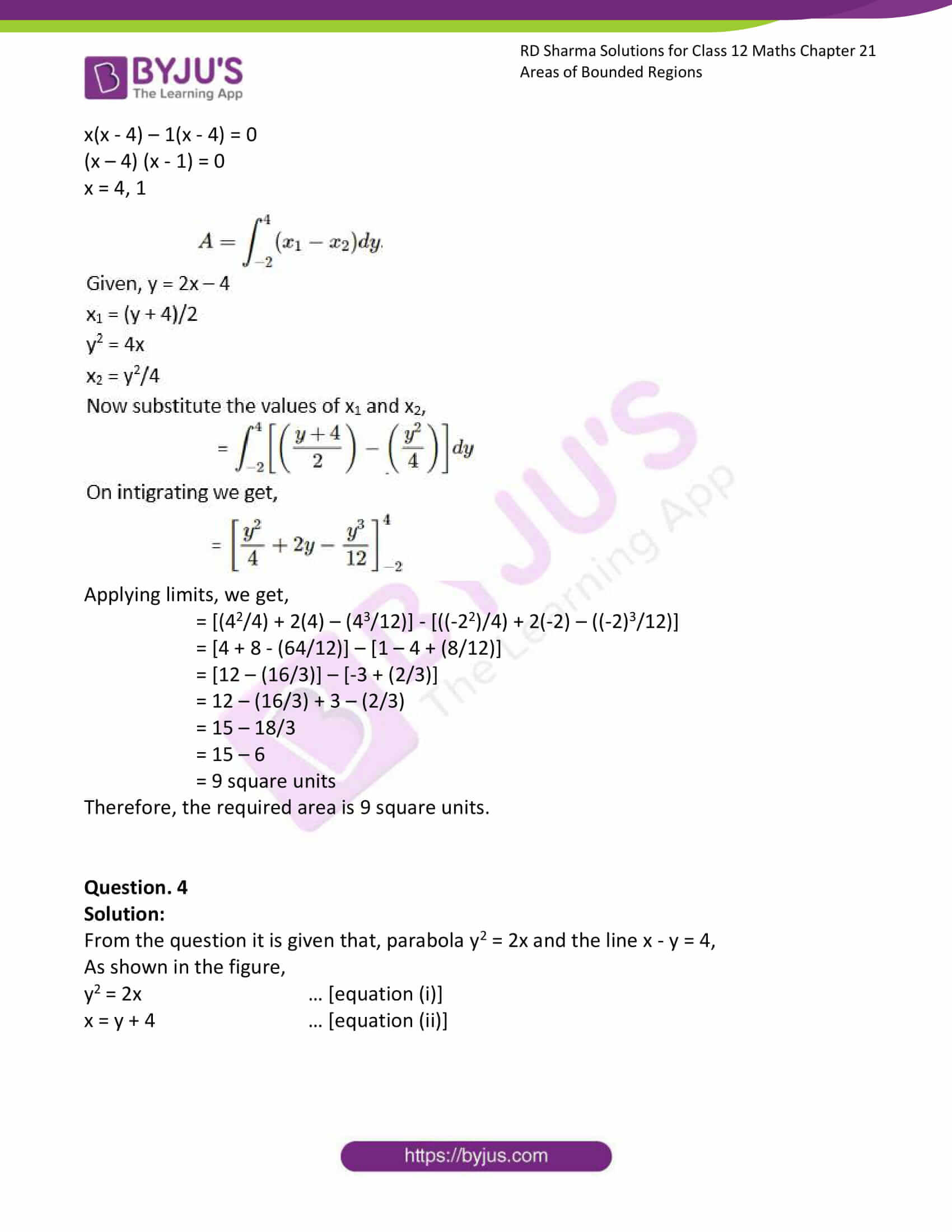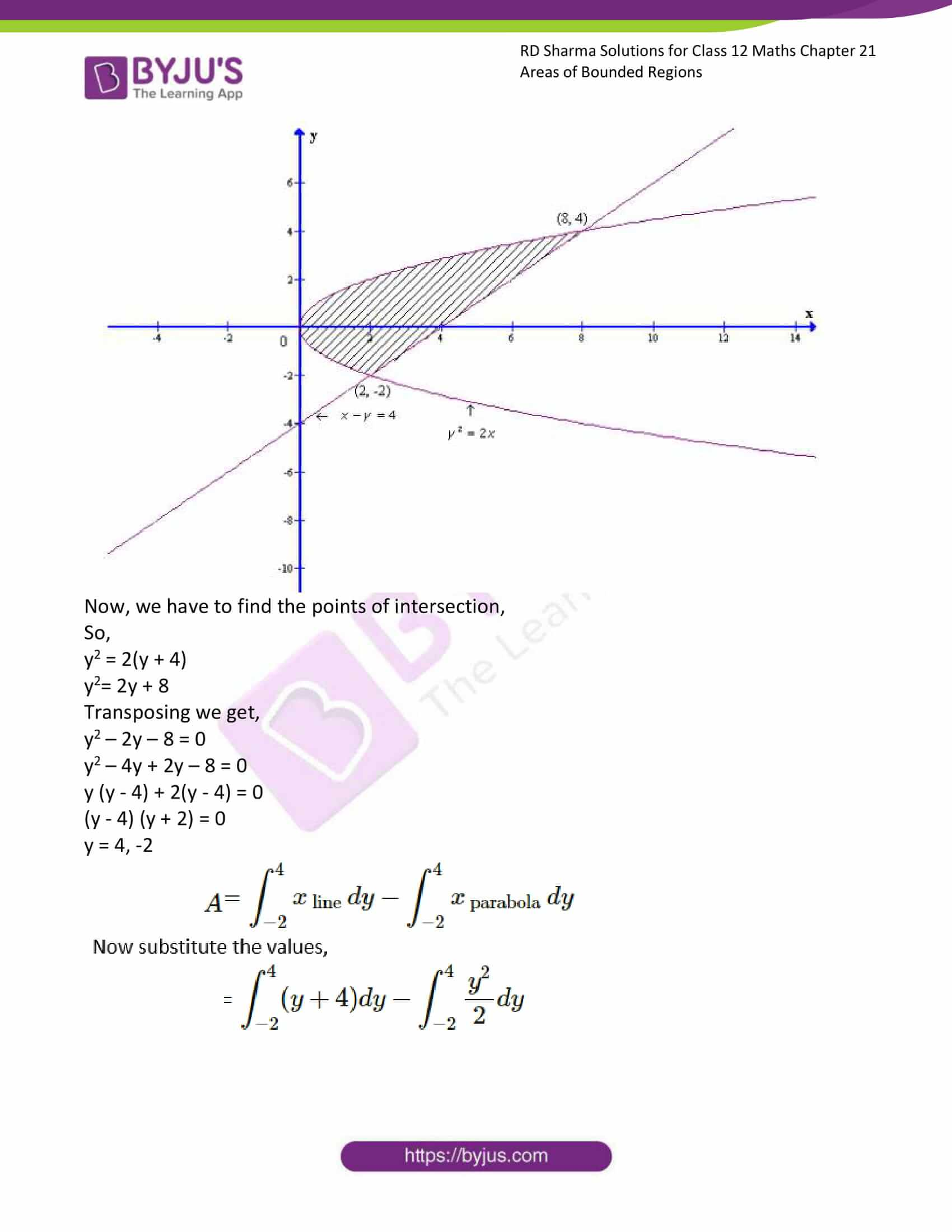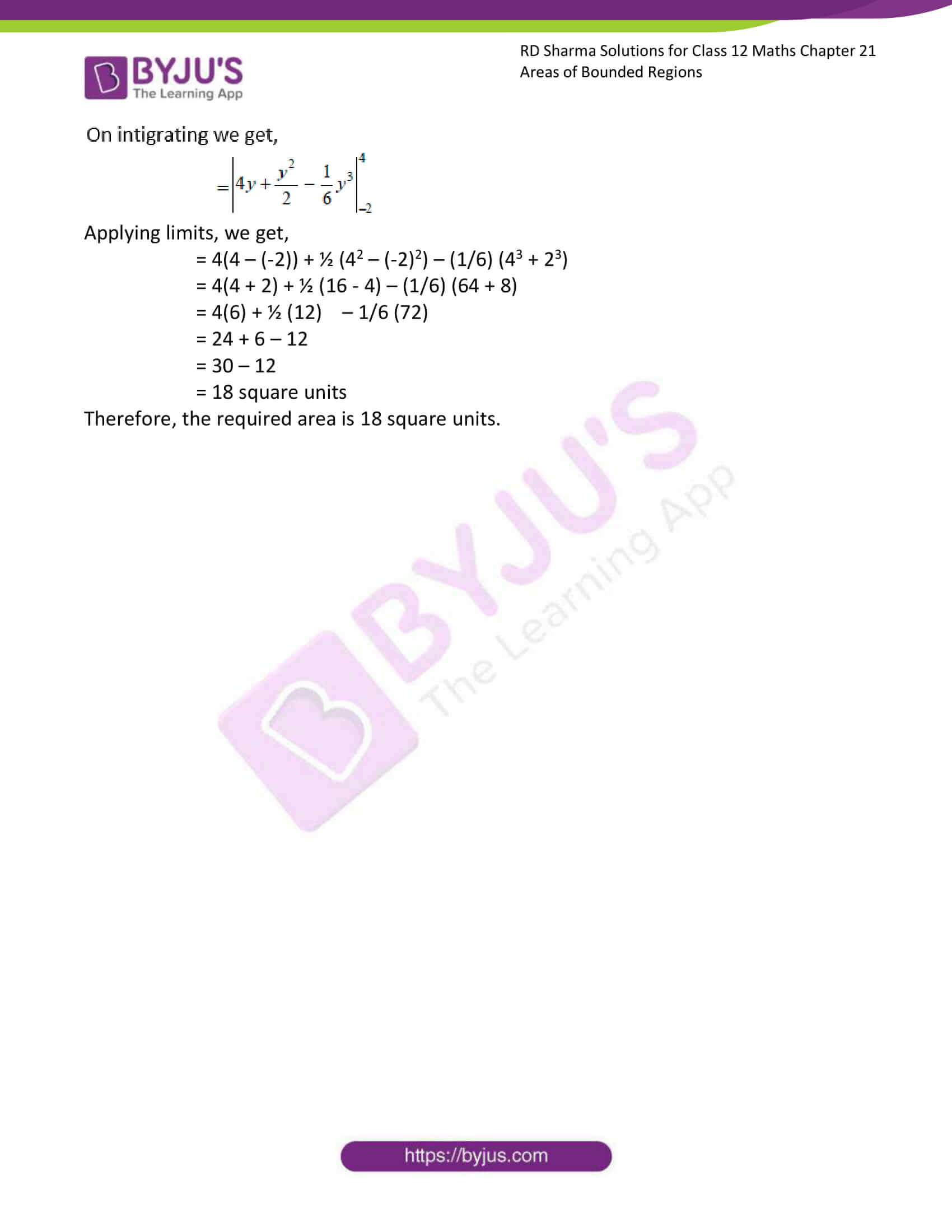### Access RD Sharma Solutions for Class 12 Maths Chapter 21 Exercise 4

EXERCISE 21.4

Question. 1

Solution:

From the question it is given that, parabola x = 4y – y2 and the line x = 2y – 3,

As shown in the figure,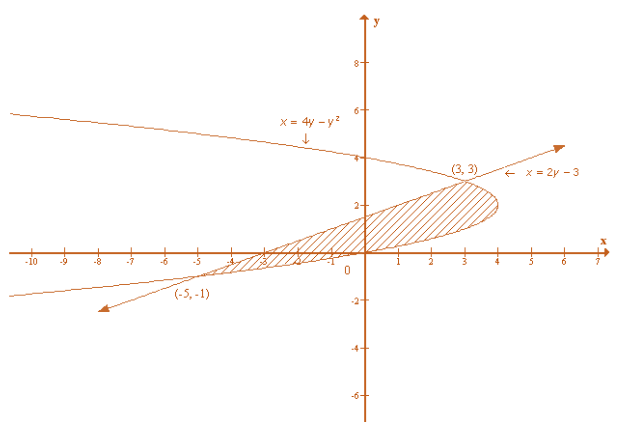x1 = 4y – y2

x2 = 2y – 3

So,

2y – 3 = 4y – y2

y2 + 2y – 4y – 3 = 0

y2 – 2y – 3 = 0

y2 – 3y + y – 3 = 0

y(y – 3) + 1(y – 3) = 0

(y – 3) (y + 1) = 0

y = -1, 3

Now, we have to find the area of the bounded region,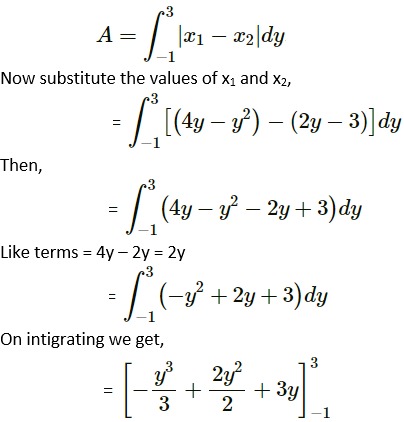Applying limits, we get,

= [- (33/3) + 2(32)/2 + 3(3)] – [- ((-1)3/3) + 2(-12)/2 + 3(-1)]

= [- 32 + 32 + 9] – [(1/3) + 1 – 3]

=  – [(1/3) – 1 + 3]

= 9 – (1/3) + 2

= 11 – (1/3)

= (33 – 1)/3

= 32/3 square units

Therefore, the required area is 32/3 square units.

Question. 2

Solution:

From the question it is given that, parabola x = 8 + 2y – y2 and the line y = – 1, y = 3

As shown in the figure,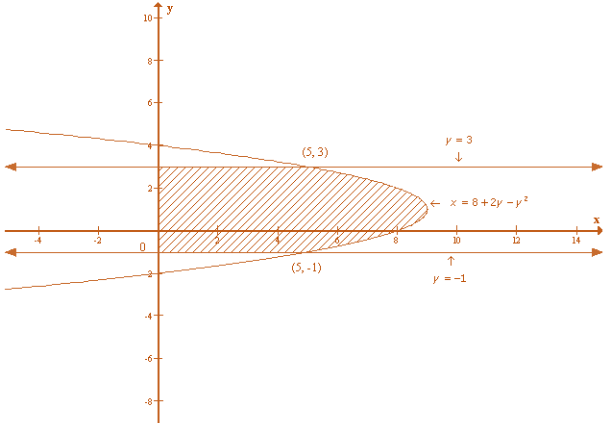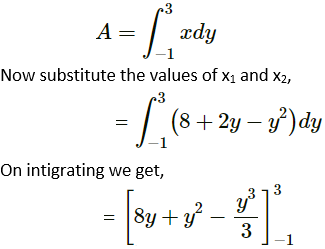Applying limits, we get,

= [8(3) + (32) – (3)3/3] – [8(-1) + (-12) – (-1)3/3]

= [24 + 9 – 9] – [-8 + 1 + (1/3)]

=  – [-7 + 1/3]

= 24 + 7 – (1/3)

= 31 – (1/3)

= (93 – 1)/3

= 92/3 square units

Therefore, the required area is 92/3 square units.

Question. 3

Solution:

From the question it is given that, parabola y2 = 4x and the line y = 2x – 4,

As shown in the figure,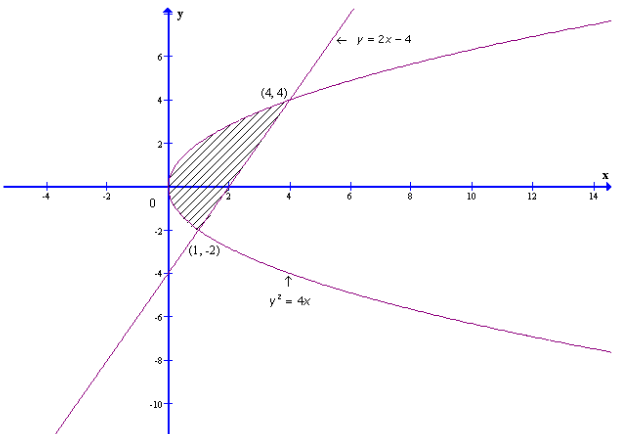So,

Now, we have to find the points of intersection,

2x – 4 = √(4x)

Squaring on both side,

(2x – 4)2 = (√(4x))2

4x2 + 16 – 16x = 4x

4x2 + 16 – 16x – 4x = 0

4x2 + 16 – 20x = 0

Dividing both side by 4 we get,

x2 – 5x + 4 = 0

x2 – 4x – x + 4 = 0

x(x – 4) – 1(x – 4) = 0

(x – 4) (x – 1) = 0

x = 4, 1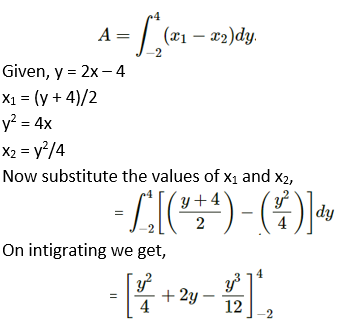Applying limits, we get,

= [(42/4) + 2(4) – (43/12)] – [((-22)/4) + 2(-2) – ((-2)3/12)]

= [4 + 8 – (64/12)] – [1 – 4 + (8/12)]

= [12 – (16/3)] – [-3 + (2/3)]

= 12 – (16/3) + 3 – (2/3)

= 15 – 18/3

= 15 – 6

= 9 square units

Therefore, the required area is 9 square units.

Question. 4

Solution:

From the question it is given that, parabola y2 = 2x and the line x – y = 4,

As shown in the figure,

y2 = 2x … [equation (i)]

x = y + 4 … [equation (ii)]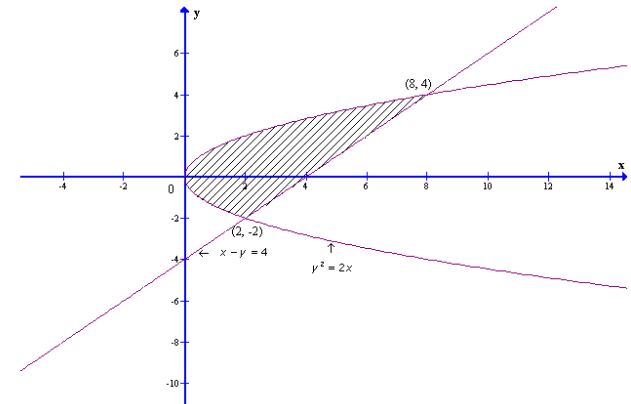Now, we have to find the points of intersection,

So,

y2 = 2(y + 4)

y2= 2y + 8

Transposing we get,

y2 – 2y – 8 = 0

y2 – 4y + 2y – 8 = 0

y (y – 4) + 2(y – 4) = 0

(y – 4) (y + 2) = 0

y = 4, -2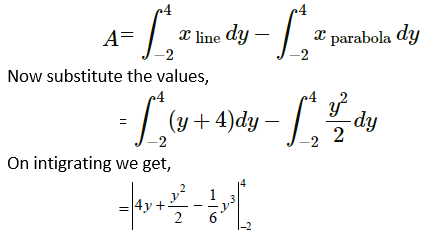Applying limits, we get,

= 4(4 – (-2)) + ½ (42 – (-2)2) – (1/6) (43 + 23)

= 4(4 + 2) + ½ (16 – 4) – (1/6) (64 + 8)

= 4(6) + ½ (12) – 1/6 (72)

= 24 + 6 – 12

= 30 – 12

= 18 square units

Therefore, the required area is 18 square units.